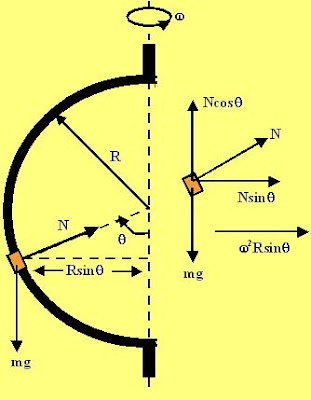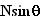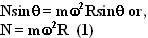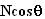## Thursday, December 13, 2007

### Irodov Problem 1.110There are two forces acting on the sleeve at any time i) the force of gravity and ii) the normal reaction from the semi-circular rod as shown in the figure. Since the sleeve is rotating about the axis indicated by the dotted line it is subject to centripetal acceleration directed towards the axis. When the sleeve is at an anglewith the vertical, the radius of the circle in which the sleeve rotates isthe centripetal acceleration is thusdirected towards the axis as depicted in the figure.

Forces acting long the horizontal plane There is only one force acting on this plane and is the component of normal reactionand this is responsible for the centripetal acceleration and so we have,Forces acting in the vertical place There are two forces acting in the vertical plane, i) the force of gravity mg and ii) the component of normal reaction. If the particle is static there will be no acceleration in this direction. Hence we have,Note that cosine can never be greater than 1, this means that for there to be an equilibrium position in the middle of the ringotherwise the ring will slip all the way to the bottom of the ring where the normal reaction will be vertical and support its entire weight.

In fact the whenthe sleeve will experience sustained stable oscillations. For a small angular disturbancethe sleeve will experience simple harmonic oscillations about the equilibrium point given by the equation,